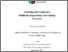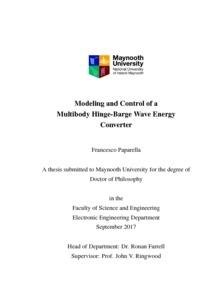# Modeling and Control of a Multibody Hinge-BargeWave Energy Converter

Paparella, Francesco (2017) Modeling and Control of a Multibody Hinge-BargeWave Energy Converter. PhD thesis, National University of Ireland Maynooth.Previewmore...Add this article to your Mendeley library

## Abstract

Wave Energy Converters (WECs) are devices used to extract energy from the waves. The particular WEC considered in this thesis is a three-body hinge-barge WEC, which is an articulated floating structure composed of 3 rectangular bodies interconnected by hinges, and it operates longitudinally to the direction to the incoming wave. The relative motion between each pair of bodies drives a Power Take-Off (PTO) system, which extracts the energy from the waves. The objective of this thesis is to increase the energy that can be extracted by a three-body hinge-barge WEC using an optimal control strategy, which computes the optimal loads applied by the PTOs driven by the relative motion between the bodies. The optimal control is formulated in the time domain, and computes the PTO loads in a coordinated way, so that the total energy extracted by the device is maximized. The optimal control strategy is formulated for a three-body hinge-barge WEC that is equipped with either passive or active PTOs. In this thesis, an optimal control strategy, for the maximization of the energy extracted by a three-body hinge-barge WEC, is derived with Pseudo-Spectral (PS) methods, which are a subset of the class of techniques used for the discretisation of integral and partial differential equations known as mean weighted residuals. In particular, PS methods based on Fourier basis functions, are used to derive an optimal control strategy, for a finite time horizon. Therefore, an optimal control strategy, with PS methods based on Fourier basis functions, cannot be applied for realtime control of the WEC, as Fourier basis functions can only represent the steady-state response of the WEC. However, PS methods based on Fourier basis functions provide a useful framework for the evaluation of the achievable power absorption performance of the WEC, with both active and passive PTOs. The Receding Horizon (RH) real-time optimal control of a three-body hingebarge WEC is derived with PS methods based on Half-Range Chebyshev-Fourier (HRCF) basis functions. The RH optimal real-time controller, with PS methods based on HRCF basis functions, maximizes the energy extracted by the WEC at each time step over a moving control horizon. In contrast to Fourier basis functions, HRCF basis functions are well suited for the approximation of non-periodic signals, allowing the representation of both the transient and steady-state response of the WEC. The optimal control strategy, with PS methods based on either Fourier or HRCF basis functions, is based on a dynamic model of the device, which is derived with two different modeling methodologies, that can be also applied to other types of multiple body WECs. The modeling methodologies are validated against wave-tank tests carried out on a 1/7th scale two-body hingebarge device, and a 1/25th and 1/20th scale three-body hinge-barge device.

Item Type: Thesis (PhD) Modeling; Control; Multibody Hinge-BargeWave Energy Converter; Faculty of Science and Engineering > Experimental Physics 9069 IR eTheses 07 Dec 2017 17:00Item control page